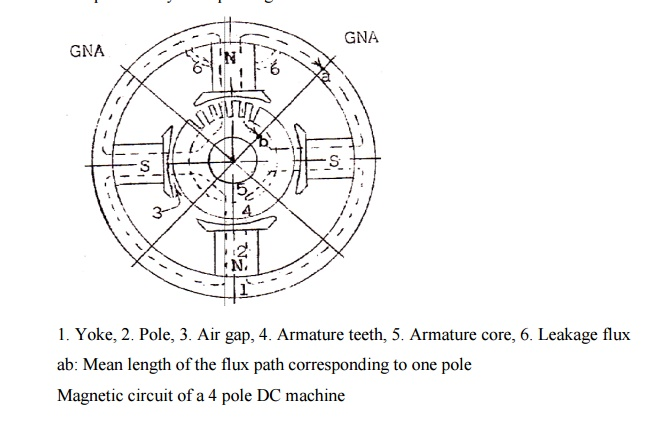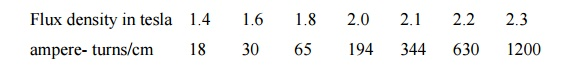Home | | Design of Electrical Machines | Magnetic circuit calculations

# Magnetic circuit calculations

The different parts of the dc machine magnetic circuit / pole are yoke, pole, air gap, armature teeth and armature core.

Magnetic circuit calculations

The different parts of the dc machine magnetic circuit / pole are yoke, pole, air gap, armature teeth and armature core. Therefore, the ampere magnetic circuit is the sum of the ampere That is,

AT / pole = ATy + ATp+ ATg1. Yoke,

2. Pole,

3. Air gap,

4. Armature teeth,

5. Armature core,

6. Leakage flux ab: Mean length of the flux path corresponding to one pole

Magnetic circuit of a 4 pole DC machine

Note: Leakage factor or Leakage coefficient LC.

All the flux produced by the pole     will not pass through the desired path i.e., air gap. Some of the flux produced by the pole will be leaking away from the air gap. The flux that passes through the air gap and cut by the armature conductors is the useful flux and that flux that leaks away from the desired path is the leakage fluxwhere LC is the Leakage factor or Leakage coefficient and lies between (1.15 to 1.25). Magnitude of flux in different parts of the magnetic circuit

a)                                                                                                 Flux in the yoke

b)                                                                                                 Flux in the pole

c)                                                                                                 Flux in the air gap

d)                                                                                                 Flux in the armature teeth

e)                                                                                                 Flux in the armature core

Reluctance of the air gapWhere

lg = Length of air gap

t = Width (pole arc) over which the flux is passing in the air gap

L = Axial length of the armature core

y t L = Air gap area / pole over which the flux is passing in the air gapPROBLEMS:

EX.1. Calculate the ampere turns required for the air gap of a DC machine given the following data. Gross core length = 40cm, air gap length = 0.5 cm, number of ducts = 5, width of each duct = 1.0cm, slot pitch = 6.5cm, average value of flux density in the air gap = 0.63T. Field form factor = 0.7, Carterâ€™s coefficient = 0.82 for opening/gap length = 1.0 and Carterâ€™s coefficient = 0.82 for opening/gap length = 1.0, and Carterâ€™s coefficient = 0.72 for opening/gap length = 2.0.

EX.2. Find the ampere-turns/pole required for a dc machine from the following data. Radical length of the air gap = 6.4mm, tooth width = 18.5 mm, slot width = 13.5mm, width of core packets = 50.8mm, width of ventilating ducts = 9.5mm, Carterâ€™s coefficient for slots and ducts = 0.27 and 0.21, maximum gap density = 0.8T. Neglect the ampere turns for the iron parts.

EX.3. Find the ampere turns required for the air gap of a 6pole, lap connected dc machine with the following data. No load voltage = 250V, air gap length = 0.8cm, pole pitch = 50cm, pole arc = 33cm,  Carterâ€™s  coefficient  for  slots  and  ducts  =  1.2,  armature  conductors  =  2000,  speed  = 300RPM, armature core length = 30cm.

EX.4. Calculate the ampere turns for the air gap of a machine using the following data. Core length = 32cm, number of ventilating ducts = 4, width of duct = 1.0cm, pole arc of ventilating ducts = 4, width of duct = 1.0cm, pole arc = 19cm. Slot pitch = 5.64 cm, semi-closed slots with slot opening = 0.5cm, air gap length = 0.5cm, flux/pole = 0.05Wb.

EX.5.          A DC machine has an armature diameter of 25cm, core length of 12cm, 31 parallel slots 1.0cm wide and 3.0cm deep. Insulation on the lamination is 8.0%. The air gap is 0.4cm long and there is one radial duct 1cm wide in the core. Carterâ€™s coefficient for the slots and the duct is 0.68.

Determine the ampere turns required for the gap and teeth if the flux density in the gap is 0.7T.

The magnetization curve for the iron is:EX.6.          Find the ampere turns/pole required to drive the flux through the teeth using Simpsonâ€™s rule with the following data: flux/pole = 0.07Wb, core-length = 35cm, number of ducts = 4, width of each duct = 1.0cm, slot pitch at the gap surface = 2.5cm, slot pitch at the root of the tooth = 2.3cm, dimensions of the slot = 1.2cm x 5cm, slots/pole-pitch = 12

EX.7. Find the ampere turns required to drive the flux through the teeth with the following data using graphical method. Minimum tooth width = 1.1cm, maximum tooth width = 1.5cm, slot depth = 4.0cm, maximum value of flux density at the minimum tooth section = 2.0T. Material used for the armature is Stalloy.

EX.8. Calculate the apparent flux density at a section of the tooth of the armature of a DC machine with the following data at that section. Slot pitch = 2.4cm, slot width = 1.2 cm, armature core length including 5 ducts each 1.0cm wide = 38cm, stacking factor = 0.92, true flux density in the teeth at the section is 2.2T for which the ampere turns/m is 70000.

EX.9. Calculate the apparent flux-density at a particular section of a tooth from the following data. Tooth width = 12mm, slot width = 10mm, gross core length = 0.32mm, number of ventilating ducts = 4, width of the duct each = 10mm, real flux density = 2.2T, permeability of teeth corresponding to real flux density = 31.4x10-6H/m. Stacking factor = 0.9.

EX.10. The armature core of a DC machine has a gross length of 33cm including 3 ducts each 10mm wide, and the iron space factor is 0.9.If the slot pitch at a particular section is 25 mm and the slot width 14mm, estimate the true flux density and the MMF/m for the teeth at this section corresponding to an apparent flux/density of 23T. The magnetization curve data for the armature stamping is,Study Material, Lecturing Notes, Assignment, Reference, Wiki description explanation, brief detail
Design of Electrical Machines : DC Machines : Magnetic circuit calculations |